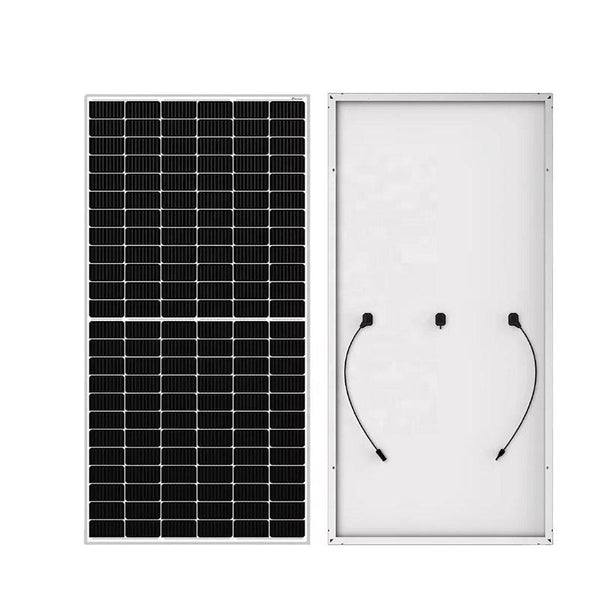# How to calculate the power of a solar panel by knowing its size?Firstly, let's first understand the standard test conditions STC (Standard Test Conditions) for solar panels, which indicate industry-wide standards for photovoltaic module performance. The specified temperature is 25 ° C (laboratory data, outdoor may have some deviation), solar irradiance is 1000W/m7, and AM 1.5 (AM represents air quality, i.e. the thickness of the atmosphere. In the equator, air quality is 1, and in Europe it is about 1.5). How can we accurately test the power of solar panels?
After understanding the above knowledge points, I will directly hand you a simple formula:
Estimated solar panel power=surface area [length (cm) * width (cm)] * 0.1 * conversion efficiency
As mentioned earlier, the conversion efficiency of mass produced single crystals is generally around 19-20%, and the calculation value is generally around 19%; The conversion efficiency of polycrystalline materials ranges from 17% to 19%, with a calculated value of around 18%. The conversion efficiency of Sunpower is between 21% and 22%, with a value of around 21% (depending on the actual conversion efficiency).
According to the above formula, the general value will be higher than the power of a normal solar panel. Why? Yesterday we mentioned that solar panels have edges. Science popularization: Why do solar panels need to have edges? Solar panel power generation is mainly achieved through the middle solar panel, so the edge of the solar panel cannot generate electricity. In summary, the power generated by solar panels is actually the power of the battery cells inside, so we can extend another formula:
Estimating the power of a solar panel=surface area of a single cell [length (cm) * width (cm)] * 0.1 * conversion efficiency * number of cells (inside the solar panel). Generally, this calculation is relatively accurate, and there may be some losses during the production process, about 5% (depending on whether there are wires or not). You can use these two methods to calculate whether the solar panel at home is this value.